# Complex Numbers

Complex numbers are extended number system. The motivation behind complex number is that there is no solution to negative roots. Consider the equation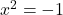, there is no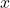that can satisfy this equation.

### Powers Of Imaginary Number i

Therefore, the imaginary numberis introduced as the solution to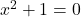.

\begin{aligned}
The \hspace{1mm}imaginary \hspace{1mm} number \hspace{1mm} i = \sqrt{-1}, where \hspace{1mm} i^2 = -1
\end{aligned}

Now, it is possible to find square root of any negative number.

Example #1

Find the square root of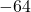.

Solution:

The square root of a negative number is possible using complex number .

\begin{aligned}
&\sqrt{-64} = \sqrt{(-1) \cdot 64}\\ \\
&= \sqrt{-1} \cdot \sqrt{64}\\ \\
&= i \cdot 8\\ \\
&\therefore \sqrt{-64} = 8i
\end{aligned}

There are few things to note here, firstly, the product property of square roots does not apply for negative numbers, meaning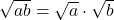only works when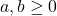. Since, we are able to use an imaginary number, it is fine to use the product property of square roots.

Secondly, you must write imaginary numberto the left of any radical. For example,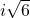is the right way, and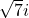will create confusion. You can write imaginary numberto the right of a real number. For example,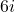.

### What is a complex number ?

Complex number is extended number system. In a complex number , there are two parts – real part and imaginary part. Therefore, real numbers are subset of complex numbers as shown in the following figure.

A standard form of complex number is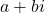where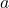is real number and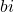is imaginary part of the complex number.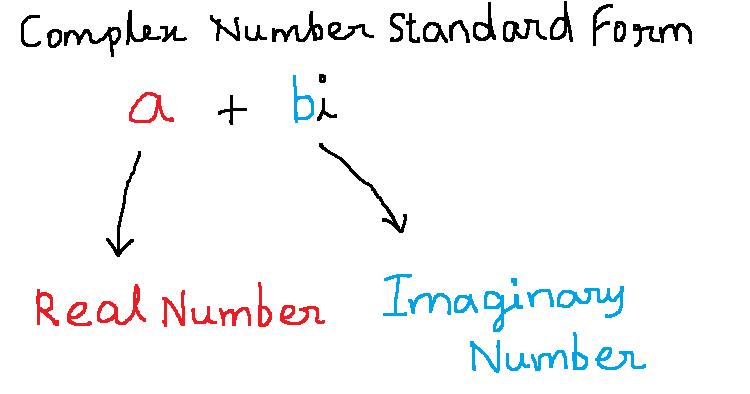Figure 2 – The standard form of complex number has two parts – real and imaginary

Real Part Of Complex Numbers

Any real number is a complex number of the formwhere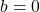. We can rewrite this complex number as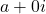.

Imaginary Part Of Complex Numbers

The imaginary part is called pure imaginary number when the real number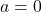, therefore, we can write the pure imaginary numbers as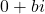.

### Equality Of Complex Numbers

Complex numbers are equal if their real part and imaginary part are equal. Supposeand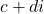are two complex numbers, then

\begin{aligned}
&a + bi = c + di\\ \\
&If \hspace{2mm} and\hspace{2mm} only \hspace{2mm} if \\ \\
&a = c \hspace{2mm}  and\hspace{2mm}  b = d\\
\end{aligned}

### Adding And Subtracting Two Complex Numbers

To add complex number is simple because you need to add the real parts and imaginary parts of two or more complex numbers separately. Ifandare two complex numbers, then their addition is

\begin{aligned}
&(a + bi) + (c + di) = (a + c) + (b + d)(i)\\
\end{aligned}

Similarly, subtracting two or more complex number requires us to subtract the real parts and the imaginary parts separately. Ifandare two complex numbers in standard form, their subtraction is

\begin{aligned}
&(a + bi) - (c + di) = a + bi - c - di\\ \\
&=(a -c) + (b - d)(i)
\end{aligned}

We will look at some examples to understand this better.

Example #2

Add the following complex numbers and write results in standard form: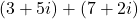.

Solution:

\begin{aligned}
&(3 + 5i) + (7 + 2i)\\ \\
&=(3 + 7) + (5 + 2)(i)\\ \\
&=10 + 7i
\end{aligned}

Example #3

Subtract the following complex numbers and write results in standard form: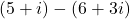.

Solution:

\begin{aligned}
&(5 + i) - (6 + 3i)\\ \\
&(5 - 6) + (1 - 3)(i)\\ \\
&-1 + (-2)(i)\\ \\
&-1 -2(i)
\end{aligned}

### Multiplying Complex Numbers

Multiplying two complex number is similar to multiplying any algebraic expression except that you have to remember the powers of “iota”. Supposeandare two complex numbers then their multiplication is

\begin{aligned}
&(a + bi)(c + di) = ac + adi + bci + bd(i \cdot i)\\ \\
&= ac + (ad + bc)i - bd\\ \\
&=ac - bd + (ad + bc)i
\end{aligned}

The real terms and the imaginary terms are multiplied separately. This results in square of “iota” in one of the terms which is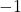.

Therefore, multiplication of two complex number results in another complex number.

There are two types of multiplication methods for complex numbers. First is multiplication by distribution method and second is multiplication as you multiply binomials( using FOIL method).

Example #4 : Distribution method

Multiply the following: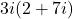.

Solution:

\begin{aligned}
&3i ( 2 + 7i ) = 3i \cdot 2 + 3i \cdot 7i\\ \\
&= 6i + 21i^2\\ \\
&= 6i + 21(-1) \hspace{1 cm} i^2 = -1\\ \\
&= 6i - 21\\ \\
&= -21 + 6i
\end{aligned}

In the above example, the term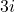is distributed to terms inside of the parentheses. Also, the result is a complex number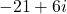which we write in standard form.

Example #5 : FOIL method

Multiply the given complex numbers using FOIL( first, outside, inside, last) method: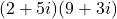.

Solution:

\begin{aligned}
&(2 + 5i)(9 + 3i) = 2 \cdot 9 + 2 \cdot 3i  + 5i \cdot 9 + 5i \cdot 3i \\ \\
&= 18 + 6i + 45i + 15i^2 \\ \\
&= 18 + (6 + 45)i + 15 (-1) \hspace{1 cm} i^2 = -1 \\ \\
&= 18 + 51i - 15\\ \\
&= 18 - 15 + 51i\\ \\
&= 3 + 51i
\end{aligned}

Therefore, multiplication by both method- distribution and FOIL result in a complex number when we multiply any two or more complex numbers. Another intresting feature of multiplication of complex number is complex conjugate, about which you will learn in the next section.

### Complex Conjugate

The complex conjugate of complex numberis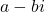, similarly, complex conjugate ofis.

Remember the difference of square in algebra, where, similarly, multiplying complex conjugates will result in real numbers.

\begin{aligned}
&(a + bi)(a - bi) = a^2 -abi + abi - b^2(i^2)\\ \\
&=a^2 - b^2(-1)\\ \\
&=a^2 + b^2\\ \\
&Similarly,\\ \\
&(a -bi)(a + bi) = a^2 + b^2
\end{aligned}

Clearly, multiplication of complex conjugates results in a real number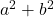.

Example #6

Find the complex conjugate and product of the complex conjugates of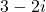.

Solution:

\begin{aligned}
&The \hspace{5px}complex \hspace{5px}conjugate \hspace{5px}of \hspace{5px}3 - 2i \hspace{5px}is \hspace{5px}3 + 2i\\ \\
&The\hspace{5px} product \hspace{5px}of \hspace{5px}complex \hspace{5px}conjugates:\\ \\
&(3 - 2i)(3 + 2i) = 9 - 4 (-1) \hspace{1 cm}\because i^2 = -1\\ \\
&=9 + 4 \\ \\
&=13\\ \\
\end{aligned}

The complex conjugates are very important because it help use to solve division involving complex numbers.

### Division of Complex Numbers

Imagine that you are dividing two complex numbers, then the goal of the division should be to obtain a real number in the denominator because we are trying to simplify the expression.

If two complex number,andare given then you must use complex conjugate of denominator to simply the expression.

\begin{aligned}
&\frac{a + bi}{c + di} = \frac{a + bi}{c + di} \cdot \frac{c - di}{c - di}\\ \\
&=\frac{ac -adi + bci  + bd}{c^2 + d^2} \\ \\
&=\frac{ac - (ad - bc)i  + bd}{c^2 + d^2}
\end{aligned}

You can see from the above example, that by multiplying with complex conjugate of denominator we get a real number in the denominator.

### Application Of Complex Number in Solving Quadratic Equations

The quadratic equation is an equation of following form: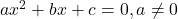where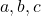are real constants. The solution of quadratic equation is given by the formula:

\begin{aligned}
&x = \frac{-b \pm \sqrt{b^2 - 4ac}}{2a}
\end{aligned}

The expression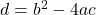is called the determinant of a quadratic equation.

• If the determinant is greater than 0,, we have real and distinct roots as solution to quadratic equation.
• If the determinant is equal to 0,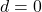, we have real and equal roots for solution.
• If the determinant is less than 0,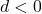, we have imaginary roots.

When the roots are imaginary , we can solve it using the complex numbers.

Example #7

Solve the following quadratic equation: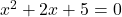Solution:

\begin{aligned}
&x = \frac{-2 \pm \sqrt{4 - 20} }{2}\\ \\
&= \frac{-2 \pm \sqrt{-16} }{2}\\ \\
&Therefore, \hspace{2mm}roots \hspace{2mm}are:\\ \\
&\frac{-2 + 4i }{2} \hspace{2mm} ,\frac{-2 - 4i }{2}
\end{aligned}

This is just one of the benefit of complex numbers, there are plenty of situations where complex number are helpful in solving, what is otherwise, not possible with only real numbers.